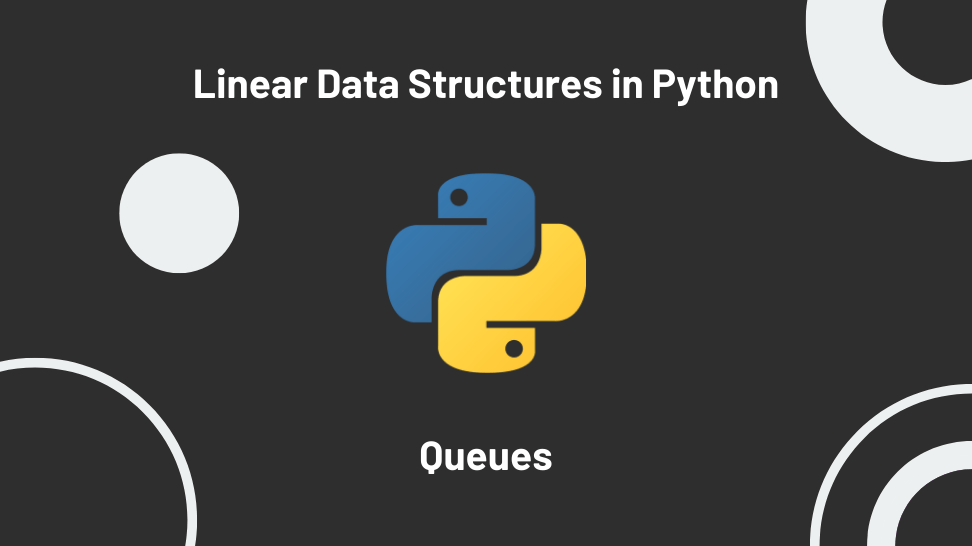In this article, I’ll explore the queue, a linear data structure we can quickly implement in Python.

This post is the second in a miniseries exploring the implementation of linear data structures. I based the series on my notes while studying for a Python technical interview.

For quick access, here is the list of posts on this series:

1. A Stack Implementation in Python
3. A Priority Queue Implementation in Python
4. Linked Lists Implementations In Python

## Introduction to queues

In computer science, a queue is an abstract data type serving as a collection of items maintained in a sequence where items are added at one end and removed at the other. The operations of a queue make it a first-in, first-out data structure (FIFO).

## Uses for queue

In programming, you can use queues to implement applications and functionality that require FIFO data access. Some examples might be:

1. The keyboard buffer.
2. The printer queue.
3. The mail queue.
4. IO buffers.

## Queue methods

Most queue implementations have the following methods:

1. queue.enqueue(x): adds an element to the back of the queue.
2. queue.dequeue(): returns and removes the element at the front of the queue.
3. queue.peek(): returns the element at the front of the queue.
4. queue.size(): returns the number of elements in the queue.
5. queue.is_empty(): returns True is the queue is empty.

## Queue implementation

Python implements multi-producer, multi-consumer queues in the Lib/queue.py module. For production applications requiring queues, you should import and use those implementations.

Since our purpose with this article is to study queues as preparation for a technical interview, we’ll do our implementation using the double-ended queue (deque) available from the collections module in Python. The reason for using deques instead of other data types like lists are:

1. In deques, appends and poplelfts are memory efficient and thread-safe.
2. In deques, appends and poplelfts have constant time complexity O(1)

Let’s go over our queue implementation using deques.

### The Queue class

We define a Queue class with a constructor that instantiates an empty deque and a __str__ method that returns a readable representation of the queue object. At the top of the module, we must import deque from collections.

### queue.is_empty():

The is_empty method returns True if the queue is empty.

This method has a constant time complexity O(1).

### queue.enqueue(item)

The enqueue method adds an item to the back of the queue, using the append method from deque.

This method has a constant time complexity O(1).

### queue.dequeue()

The dequeue method returns and removes the item at the front of the queue, using the popleft method from deque. To avoid getting an IndexError when popping from an empty queue, we first check if it is empty and return None.

This method has a constant time complexity O(1).

### queue.peek()

The peek method returns the item at the front of the queue (index 0). As with dequeue, we need to return None if the queue is empty to avoid an IndexError.

This method has a constant time complexity O(1).

### queue.size()

The size method returns the length of the queue.

This method has a constant time complexity O(1).

### The full implementation

Below is the full implementation and some test cases:

## Conclusion

In this article, we implemented a queue in Python. The queue is a linear data structure useful when accessing data in a first-in, first-out fashion. Our implementation uses deque, allowing for thread-safe, memory efficient, and constant time enqueues and dequeues.

In the next article, we’ll explore the priority queue. See you there!

## References

Wikipedia – Queue (abstract data type)

GeeksForGeeks – Applications of Queue Data Structure

Python Documentation – deque

Python Documentation – queue – A synchronized queue class